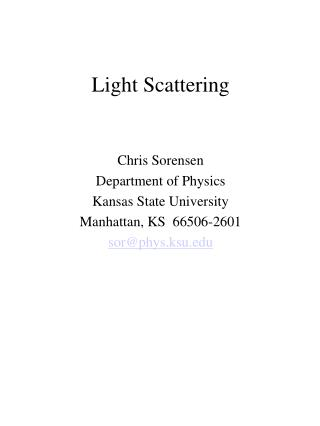DownloadDownload PresentationLight Scattering

# Light Scattering

Download Presentation## Light Scattering

- - - - - - - - - - - - - - - - - - - - - - - - - - - E N D - - - - - - - - - - - - - - - - - - - - - - - - - - -
##### Presentation Transcript

1. Light Scattering Chris Sorensen Department of Physics Kansas State University Manhattan, KS 66506-2601 sor@phys.ksu.edu

2. Light Scattering It’s how we see the world. Every non-luminous thing we see, we see via light scattering. Reflection is a special form of light scattering. Non Invasive Probe of Aerosol Systems Colloid Science Biophysics Condensed Matter Physics Aerosol Science Atmospheric Visibility Earth’s Radiation Budget Problems Becoming More Complex

3. Laser Light Scattering Typically “VU” scattering incident polarization vertical, no polarizer on detector, i.e., unpolarized.

4. Polarization by scattering

5. Rayleigh ScatteringScattering from Small Particles What do we mean by “small”? ---small compared to . (Only two length scales, R and ).

6. Rayleigh Scattering (2) Since R<<, the point sub volumes within particle  see the same incident phase  are all essentially the same distance from the detector Thus they scatter in phase to the detector (regardless of angle). Total scattering amplitude Total scattered intensity

7. Rayleigh Scattering (3)Unit Analysis Cross section units: area = (length)2. But so far we have σ ~ V2 = (length)6

8. Rayleigh Scattering (3b)Unit Analysis Cross section units: area = (length)2 . But so far we have So there must be another length scale involved—the only other length scale is . So

9. Blue sky and red sunset. Consequences of Rayleigh Scattering (1)

10. Red and blue due to Rayleigh scattering

11. Consequences of Rayleigh Scattering (2) • Isotropic scattering

12. Consequences of Rayleigh Scattering (3)The Tyndall Effect The increased scattering as a system coarsens, e.g., precipitates. Is N  N V2/4 Hence Is  Vparticle.

13. Single Slit Diffraction Patterns a = slit width,  = light wavelength

14. The scattering wave vector, q. Theory leads to q = (4π/λ)sin(θ/2) Much more useful than the scattering angle, θ.

15. Rayleigh-Debye-Gans Theory  [3u-3 (sin u-u cosu)]2 Where u = qR (a nice dimensionless variable!) Simply the square of the Fourier Transform of a sphere. Good when  = 2kR|m-1|<1 where m is the particle refractive index and is the phase shift parameter.

16. RDGPlotted vs theta

17. RDGPlotted vs. qR

18. RDG • RDG is equal to Rayleigh when scattering angle is small in the “Forward Lobe” • RDG contains Porod’s Law when qR>1 I  q-4

19. Inverse q Note that since q = (4π/λ)sin(θ/2), inverse q has units of length. Inverse q is the “length scale of the scattering experiment”.

20. Crossovers and Length Scales. On log-log plots one finds in general that I(q) vs. q crosses over from one slope to another (i.e., from one power law to another) when q passes through a length scale of the scatterer as demonstrate here.

21. How big are the water drops in the fog? Why is the edge of the halo red?

22. Lunar halo

23. Mie Scattering for a Sphere

24. e.g., if (green), kR=1 implies R=0.08 (Dia=0.16 ). Mie Scattering for Spheres m=1.33 Various kR = (2π/λ)R

25. Patterns in Mie Scattering(normalized to I(0))Sorensen and Fischbach, Opt. Commun. 173, 145 (2000).

26. Quasi Universality with 

27. Generic Features of Mie Scattering

28. The Mie Ripples Δθ = λ/diameter Useful for large, single particles or very narrow size distribution

29. Forward scattering from large (10μ) particles

30. Effect of polydispersity on the ripples

31. Total Scattering Cross Section

32. Fractal Aggregate Rg = Radius of Gyration a root-mean-square radius N=monomers/aggregate N ~ RgD D = Fractal dimension

33. Fractal Aggregate Scattering Notation: Dm=Df=D No ripples because surface is “soft”.

34. Guinier Analysis When Thus Regardless of shape Regardless of refractive index Plot I(0)/I(q) vs. q2 Slope = Rg2/3 (Recall the Zimm plot of biophysics)

35. Classic Zimm Plot cellulose nitrate fraction in acetone (Benoit, Holtzer, and Doty, JPC58, 635 (1954).

36. Premixed CH4/O2 Flame Soot Gangopadhyay et al. Appl. Optics 30, 4859 (1991).

37. Kim, Sorensen and Chakrabarti, Langmuir 20, 3969 (2004).

38. Tyndall Effect for Fractal Aggregate

39. Baby Bomb

40. Scattered Light Intensity vs. q

41. Shearing 1 min after the onset of aggregation. Shear rate: 2.3 sec-1. Gel time: 50 ± 10 min.